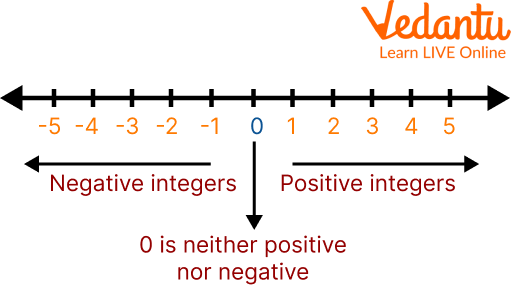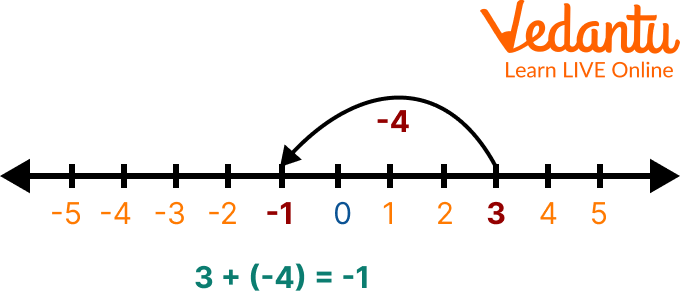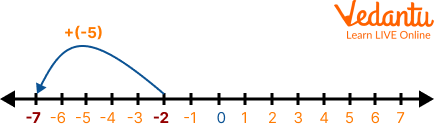Courses
Courses for Kids
Free study material
Offline Centres
More

# Representation of Integers on Number LineLast updated date: 05th Dec 2023
Total views: 116.4k
Views today: 3.16k## Learning How to Represent Integers on the Number Line!

Integers are a set of positive and whole numbers including 0. A number line is a visual representation of numbers on a straight line. This line is used to compare numbers that are placed at equal intervals on an infinite line that extends on both sides, horizontally or vertically. It’s a helpful tool for students wanting to learn the number system. This article will help you master the number line and may help you in your number line project.

## What is the Number Line?

A one-dimensional graph of a line in which integers are shown as specially marked dots that are evenly spaced is called a number line. It is divided into two symmetrical halves by the origin, the number zero.Number Line

## Set of Integers

Integers are the set of numbers formed by all natural numbers (positive numbers) by zero and by negative numbers (those that are smaller than zero and have a minus sign in front)

• The natural numbers: $1,2,3,4,5,6,7 \cdots$ so on to infinity ( $\infty$ ) are the positive numbers. They could be written as $+1,+2,+3,+4,+5, \ldots$.

• The zero: 0 (It is neither positive nor negative, it is neutral)

• The negative numbers: $-1,-2,-3,-4,-5,-6,-7 \ldots$ so on to minus infinity $(-\infty)$

You may ask yourself, are the numbers natural numbers or integers?

Well, it is inside the two sets because they belong to the natural numbers, but this set is contained in the integers. So, in other words, the natural numbers are a subset of the integers.

The set of integers is represented by the letter $Z$:

$Z=\{\ldots-6,-5,-4,-3,-2,-1,0,1,2,3,4,5,6 \ldots\}$

## How to Represent Integers on a Number Line?

The representation of integers on number line is done in the following way:

1. We draw the line, divide into equal parts and put 0 in the centre.A Line

2. We place the positive numbers to the right of zero, increasing their value by one unit, from left to right.

3. Now we place the negative numbers to the left of zero. They all have a minus sign in front of them.The Number Line

This can be clearly understood with the help of a number line. Now, after seeing how to represent integers on the number line, we are going to see how they are ordered.

## Number Line Addition and Subtraction of Integers

Now that we understand how to add and subtract integers on a number line of integers, we can learn other arithmetic operations. To add a positive number means that we move the point to the right of the number line. Similarly, to add a negative number implies that we move the point to the left of the number line.Number Line Addition and Subtraction of Integers

## Multiplication of Integers on Number Line

The application of a multiplication operation on a given set of integers on a number line is known as multiplication of integers on a number line. Repeated addition is another name for multiplication. To conduct multiplication on a number line, we begin at zero and work our way to the right side of the number line for a certain number of times. Consider the following example. Using a number line, multiply 3 5 times.

On the number line, three sets of five identical intervals must be made beginning at zero. We will achieve 15 by organising three separate groups. Consider the following number line: 3 x 5 = 15.Multiplication of Numbers on Number Line

Now let’s see some number line examples.

### Solved Examples

Q 1. Perform the operation 3 + (-4) using a number line.

Ans:Here, since the second number is $-4$ and the result is $-1$, we start with 3 and move 4 units to the left on the number line.

Q 2. How will you operate 7 + 12 using a number line? Explain in words.

Ans: The number 7 is marked on the number line. From there, we will proceed 12 units to the right on the number line, where we will eventually arrive at 19.

As a result, we use a number line to calculate 7 + 12 = 19.

Q 3. Add -5 and -2 using the number line.

Ans: Here, the first number is -5 and the second number is -2; both are negative. Locate -2 on the number line. Then moving 5 places to the left will give -7.Q 4. Subtract 5 from 2 using the number line

Ans: Here the first number is 2 and the second number is 5; both are positive. First, locate 2 on the number line. Then moving 5 places to the left will give us -3.Subtraction on Number Line

### Practice Questions

Q 1. When -2 is added to 8, keeping 8 as the reference, we move by ____ on the number line.

Ans: 2 units towards the left

Q 2. Which way do we move on the number line when we add a positive number?

Ans: Right of the number line

Q 3. Which way do the negative numbers are placed to the zero?

Ans: To the left

Q 4. Which way do the positive numbers lie to the zero?

Ans: To the right

Q 5. The addition of 8 and 1 in the number line is____

Ans: 9

## Summary

Integers are plotted on a number line by adhering to the following fundamental guidelines: all positive numbers higher than zero, like $1,2,3, \ldots$, etc., are plotted to the right of zero, while all negative integers less than zero, like, $-1,-2,-3, \ldots$ etc., are plotted to the left of zero. The integer zero is precisely displayed in the middle. As an illustration, the integer 3 is plotted to the right of 0 , whereas the value $-4$ is drawn to the left of 0.

## FAQs on Representation of Integers on Number Line

1. What is the number line in fraction?

A fraction on a number line is a graphic representation of fractions. It is accomplished by plotting the given fraction(s) on a number line where the section or distance between two integers is divided into equal portions equal to the given fraction's denominator.

2. Can whole numbers be represented on a number line?

Yes, whole numbers can be represented on a number line.

3. Who created the number line?

The concept of the number line was developed by Napier (1616). John Wallis introduced the number line into his algebra.